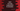# For loop in dart : explanation with example# Introduction :

You will find for loop in almost all programming languages. The syntax of a for loop is almost the same. The for loop runs for a specific number of times. It checks one condition and keeps iterating through the code in the loop until the condition is true. In this tutorial, we will learn how to use a for loop in dart with examples.

for loop can be used in many cases like iterating through the array elements, iterating through the characters of a string, iterating through the list items etc. Actually, for loop can be used with any fixed set of items. Let’s take a look at the syntax of the for loop in dart:

## Syntax of for loop :

The syntax of the for loop is as below :

``````for(initial_counter_value, loop_condition,step_changes){
//statements
}``````

Here, initialcountervalue is the starting value of the counter or flag that we will use in the loop. loopcondition_ is the condition of the loop that will be checked on each iteration of the loop. The for loop will run until this condition is true. stepchanges_ is the changes for the counter variable on each iteration. We will change the counter variable on each iteration.

For example,

``````for(var i=0;i<10;i++){
//statements
}``````

This for loop will run for 10 times.

For the 1st iteration, the value of i is 0 i.e. the starting value. For the 2nd iteration, the value of i is 1. It is increased by 1 because we have one i++ as the stepchanges. For the 3rd iteration, the value of _i is 2. Similarly, it will run up to 10th iteration with i value 9. For the 11th iteration, it will not execute because the value of i will become 10 and as per our condition, i should be always less than 10.

Let me show you a couple of more examples to make this thing clear :

## Example 1: For loop with one increment :

``````void main() {
for (var i = 0; i < 10; i++) {
if(i%2 == 0){
print(i);
}
}
}``````

This program will increment the value of i by 1 on each step and for each value, it will check if it is divisible by 2 or not. Basically, this program will print out all one-digit even numbers.

## Example 2: For loop with 3 increments:

You can set any condition to the counter increment step. The below example will increment the value i by 3 on each step.

``````void main() {
for (var i = 0; i < 20; i=i+3) {
print(i);
}
}``````

## Example 3: For loop with one decrement :

You can also write decrementing counters in a loop. In the below program, the value of i is decreasing by 1 on each step.

``````void main() {
for (var i = 10; i > 0; i--) {
print(i);
}
}``````

### Conclusion :

In this tutorial, we have learned how to use for loop in dart. Try to run the above examples and get comfortable with it. We will post a few more examples on for loop soon. Don’t forget to subscribe to our newsletter and don’t hesitate to drop one comment below if you have any queries.